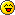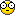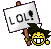First Steps...First Steps...

Here are the first in a long series of Multiple Choice practice problems: Write up a solution for your answer, when the person answers correctly, I will let you folks know.

*NOTE: ALL Multiple Choice questions, are NON CALCULATOR ITEMS! For simplicity, all questions that use acceleration due to gravity will be 10 m/s2 NOT 9.8m/s2.

MC 1) A firework is shot straight up in the air with an initial speed of 50 m/s. What is the maximum height it reaches?
(A) 12.5 m
(B) 25 m
(C) 125 m
(D) 250 m
(E) 1250 m

MC 2) On a strange airless planet, a ball is thrown downward from a height of 17 m. The ball initially travels at 15 m/s. If the ball hits the ground in 1 s, what is this planet's gravitational acceleration?
(A) 2 m/s2
(B) 4 m/s2
(C) 6 m/s2
(D) 8 m/s2
(E) 10 m/s2

FR 1) An airplane attempts to drop a bomb on a target. When the bomb is released, the plane is flying upward at an angle of 30° above the horizontal at a speed of 200 m/s. At the point of release, the plane's altitude is 2.0 km. The bomb hits the target.
(a) Determine the magnitude and direction of the vertical component of the bomb's velocity at the point of release.

(b) Determine the magnitude and direction of the horizontal component of the bomb's velocity at the point when the bomb contacts the target.

(c) Determine how much time it takes for the bomb to hit the target after it is released.

(d) At the point of release, what angle below the horizontal does the pilot have to look in order to see the target?Supreme Math GOD

Posts : 22
Join date : 2009-03-19
Age : 37Re: First Steps...

MC 1)
Vf2=V02+2aX
02=502+2(-10)(X)
0=2500-20X
X=125

MC 2)
X=V0t+(1/2)at2
-17=-15+.5a
a=-4

FR 1)
a) Vy=VsinΘ
Vy=(200 m/s)sin(30°)=100 m/s

b)V0x remains constant
V0x=V0cosΘ
V0x=(200 m/s)cos(30°)=173170 m/s

c) Y=V0yt-.5gt2
-2000 m=(100 m/s)t-.5(9.8 m/s2)t2
-4.9t2+100t+2000=0
t=32.8 s

-2000 m=(100 m/s)t-.5(-10 m/s2)t2
-5.0t2+100t+2000=0
t=32 s

d)X=Vxt
X=(173 m/s)(32.8 s)=5681 m
Y=-2000 m

tan Θ=5681/2000
Θ=tan-1(5681/2000)=70.61°

Θ=tan-1 5681/-2000
Θ=-70.61° (70.61° below the horizontal)

Last edited by jodo_059 on Tue Jun 22, 2010 12:32 am; edited 1 time in totaljodo_059

Posts : 11
Join date : 2010-06-02Re: First Steps...

Well done. A few things to point out though:
(1) on the AP exam, unlike what we use in the homework assignments acceleration due to gravity is -10 not 9.8!

(2) In part (b) of FR 1, sig figs would tell us that the answer would be 170 m/s.

(3) FR 1 (d) read carefully, the problem says at what angle does the PILOT have to look down. Does the pilot look down at an angle of 70 degrees?Supreme Math GOD

Posts : 22
Join date : 2009-03-19
Age : 37Re: First Steps...

For the first multiple choice question, point D is the highest point. There should be no y direction acceleration, and since the rock is moving towards right, the net acceleration would be to the right?

jingtaiwu

Posts : 5
Join date : 2010-06-10Re: First Steps...

For question 2...
I'm guessing the grid lines are there so you have some sort of "unit" to use.
So...

Fy=Fnormal+Fy-applied+Fgravity=(2)+(2)+(-4)=0
No movement in the y-directions (A and D are wrong)

Fx=Fx-applied+Ffriction=(4)+(-4)=0
No movement in the x-directions either...jodo_059

Posts : 11
Join date : 2010-06-02Re: First Steps...

Shouldn't the normal force be three units? Because the tip of the arrow count as a unit, too?

jingtaiwu

Posts : 5
Join date : 2010-06-10Re: First Steps...

Fn=3
Fg=-5
Ff=5
Fay=2
Fax=5

Fy=2+3-5=0
Fx=5+-5=0

LOL, I guess that doesn't matter because you all count one unit short... NVM!~

jingtaiwu

Posts : 5
Join date : 2010-06-10Re: First Steps...

jingtaiwu wrote:Shouldn't the normal force be three units? Because the tip of the arrow count as a unit, too?
well...since the magnitudes are proportional to their lengths, i guess as long as you count from the same points it should be okay...jodo_059

Posts : 11
Join date : 2010-06-02Re: First Steps...

Are you folks sure...if you are then what would you folks say your answers are?Supreme Math GOD

Posts : 22
Join date : 2009-03-19
Age : 37Re: First Steps...

for the force question,
so the box is on a horizontal surface and Fgravity is greater than Fnormal so its resting on the horizontal surface.

so now the questions is is it going to the left or staying still?
well Fx = Fappliedcos&Theta; - Ffriction = ma
and Fappliedcos(theta) = 5 and Ffriction = 5
and 5 - 5 = 0 = ma. therefore there is no accelleration SO THE BOX ISN'T MOVING!shakalohana

Posts : 17
Join date : 2010-06-04Re: First Steps...

and for the rock one,
at point D, its the highest point so acceleration = 0 and since it was going up, now it should be going down? so is the answer D? or is it answer E since there is no net acceleration at point D....and the other satellite astronaut one...help!shakalohana

Posts : 17
Join date : 2010-06-04Re: First Steps...

Couple of remarks:

(a) We are not using work, b/c we haven't covered it yet...problem can be solved without any math.

(b) Which one is it? (d) or (e)? Why?

(c) For the satellite problem think about the inverse square law (i.e. Fg = ?)Supreme Math GOD

Posts : 22
Join date : 2009-03-19
Age : 37Re: First Steps...

For the rock problem, the answer should be D. The rock moves at a constant velocity in the X-direction, so Ax is 0. In the Y-direction, the rock's velocity is continuously decreasing, so Ay is negative. The rock doesn't accelerate in the X-direction, but is constantly decelerating in the Y-direction, even when the rock's velocity is 0, as it is at Point D.jodo_059

Posts : 11
Join date : 2010-06-02Re: First Steps...

Yay! Good JOB!Supreme Math GOD

Posts : 22
Join date : 2009-03-19
Age : 37Re: First Steps...

ooo for the position vs. time graph asking for the velocity vs. time. it's e) because the slope of the lines along the curve get bigger and then stay constant and then get smaller and yea! xD

and for the the box problem, um is it just C) because there's all different forces working on the box? lol

and the satellite one, i don't think i learned the inverse square law...what chapter is that in the cutnell and johnson textbook?...shakalohana

Posts : 17
Join date : 2010-06-04Re: First Steps...

The box problem...hahaha nope! Caught you didn't it!!! Bet it caught all of you...the answer is E Can anyone tell me why?

Fg = G(m1m2)/R2 Which graph would represent this the best?Supreme Math GOD

Posts : 22
Join date : 2009-03-19
Age : 37Re: First Steps...

oh!! ok for the box problem, is it because it shouldn't be Force of gravity but Force of mass*gravity = weight?
riggghhttt?? haha

oh! and the velocity time graph, i meant D) lol

and for the satellite.
Fg = G(m1m2)/R2
uhhhmr k, your icon is so funny! i get distracted by it!shakalohana

Posts : 17
Join date : 2010-06-04Re: First Steps...

For the box problem...
The answer is E, because even though the forces are balanced, and the acceleration is 0, we cannot say that velocity is 0. We can only say that velocity is constant, so the box could have been moving at a constant.

....trickyjodo_059

Posts : 11
Join date : 2010-06-02Re: First Steps...

you make a point there jared lolshakalohana

Posts : 17
Join date : 2010-06-04Re: First Steps...

Very GOOD!!! BOX PROBLEM SOLVED! VELOCITY TIME GRAPH PROBLEM SOLVED!Supreme Math GOD

Posts : 22
Join date : 2009-03-19
Age : 37Re: First Steps...

ok so for the satellite how do you know if the graph should be a straight line or curved? sorry im kinda late on this conversation but i figured out how to do the other problems through everyones discussion

corynne

Posts : 1
Join date : 2010-06-10Re: First Steps...

I think since the bottom of Gm1m2/r^2 that it should be curved because the square graph itself is a porabloa so it shoudln't be any with a straight line

Tyl0r

Posts : 1
Join date : 2010-06-08
Age : 26Re: First Steps...

for the satellite problem...
answer is D. If the astronaut is inside the satellite, he won't feel any gravity.jodo_059

Posts : 11
Join date : 2010-06-02Re: First Steps...

jared, can you explain the satellite problem to me tomorrow?
we have study session tomorrow right?
hamilton at 10?

mr k. have you come back alive??shakalohana

Posts : 17
Join date : 2010-06-04Re: First Steps...